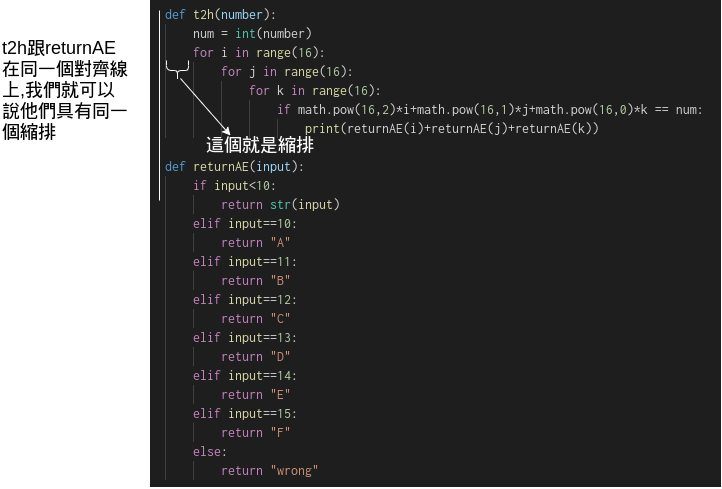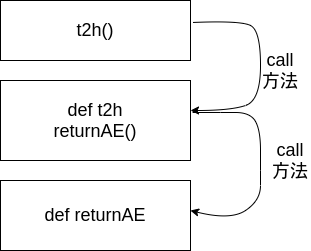#DAY 6
1
Software Development

## 寫在前面

python是一種相當適合新手學習也相當適合高手使用的語言

### python版本

python目前分為2.X跟3.X兩種版本,

python --version

## 程式碼

``````import math

# 定義開始

def t2h(number):
num = int(number)
for i in range(16):
for j in range(16):
for k in range(16):
if math.pow(16,2)*i+math.pow(16,1)*j+math.pow(16,0)*k == num:
print(returnAE(i)+returnAE(j)+returnAE(k))

def returnAE(input):
if input<10:
return str(input)
elif input==10:
return "A"
elif input==11:
return "B"
elif input==12:
return "C"
elif input==13:
return "D"
elif input==14:
return "E"
elif input==15:
return "F"
else:
return "wrong"

def h2t(number):
output = 0
pow = len(number)
i = 0
while i < pow:
output += int(AEreturn(number[i])*math.pow(16,pow-i-1))
i+=1
print(output)

def AEreturn(input):
try :
return int(input)
except:
if input=="A":
return 10
elif input=="B":
return 11
elif input=="C":
return 12
elif input=="D":
return 13
elif input=="E":
return 14
elif input=="F":
return 15
else:
return 0

# 程式開始

print("Tell me what you want to do:")
convert = input("(1)T to H (2)H to T\n")

if convert == "1":
number = input("Please enter the number\n")
t2h(number)
elif convert == "2":
number = input("Please enter the number\n")
h2t(number)
else:
print("Wrong select")

``````

python convert.py

C#從main方法當作進入點是因為C#是一種編譯式的語言,而python則是直譯式的語言

;呢? 怎麼都沒看到

python是一種不需要;的語言

### 我們先從熟悉的輸入輸出開始吧

``````print("Tell me what you want to do:")
convert = input("(1)T to H (2)H to T\n")
``````

``````\n
``````

### 邏輯判斷 if

``````if convert == "1":
number = input("Please enter the number\n")
t2h(number)
elif convert == "2":
number = input("Please enter the number\n")
h2t(number)
else:
print("Wrong select")
``````

python內是沒有switch的喔,請用if else判斷吧

python的else if 被簡寫成elifif的其他部份跟C#類似就不提拉

``````t2h(number)
``````

### 方法

``````def t2h(number):
num = int(number)
for i in range(16):
for j in range(16):
for k in range(16):
if math.pow(16,2)*i+math.pow(16,1)*j+math.pow(16,0)*k == num:
print(returnAE(i)+returnAE(j)+returnAE(k))
``````

python內定義方法的關鍵字是def

``````def 方法名稱(輸入值):
``````

### 轉型

``````num = int(number)
``````

### 迴圈

``````for i in range(16):
for j in range(16):
for k in range(16):
``````

``````for i in range(10, 1, -2):
#10 8 6 4 2

for i in range(1,10):
#1 2 3 4 5 6 7 8 9
``````

### import

``````if math.pow(16,2)*i+math.pow(16,1)*j+math.pow(16,0)*k == num:
``````

``````import math
``````

C#內我們說過using後你就可以省略using的語句

``````using System; //使用了這個之後你就可以省略System.Console.WriteLine的System了
``````

C#的Using是命名空間,而python的import是引用函式庫

``````def returnAE(input):
if input<10:
return str(input)
elif input==10:
return "A"
elif input==11:
return "B"
elif input==12:
return "C"
elif input==13:
return "D"
elif input==14:
return "E"
elif input==15:
return "F"
else:
return "wrong"
``````

``````return str(input)
``````

``````print(returnAE(i)+returnAE(j)+returnAE(k))
``````

### 迴圈while

``````def h2t(number):
output = 0
pow = len(number)
i = 0
while i < pow:
output += int(AEreturn(number[i])*math.pow(16,pow-i-1))
i+=1
print(output)
``````

``````AEreturn(number[i])*math.pow(16,pow-i-1)
``````

### 錯誤處理

python一樣有錯誤處理喔,只是語法不太一樣

``````def AEreturn(input):
try :
return int(input)
except:
if input=="A":
return 10
elif input=="B":
return 11
elif input=="C":
return 12
elif input=="D":
return 13
elif input=="E":
return 14
elif input=="F":
return 15
else:
return 0
``````

### 註解

``````# 定義開始
``````

python的單行註解是使用#

``````'''

'''
``````

print("Tell me what you want to do:")
convert = input("(1)T to H (2)H to T\n")

## 小結

• 基本結構
• 直譯式語言,程式進入點就位於程式的開頭
• 行尾不需要;
• 使用tab取代{}
• 印出/讀取
• 使用print輸出
• input輸入
• 方法的結構
• 關鍵字是def
• 動態型別,不需要提前告知輸入與輸出的型別
• 邏輯控制
• 沒有switch
• if 不需要()跟{}
• 迴圈控制
• for 有好用的for range可以用
• while 不需要(),{},但是有:
• 型別
• 動態,但是是強型別系統,沒辦法不轉型就比較字串跟數值
• 冪次計算
• math.pow()(還記得我說過直譯式語言是一行一行執行的嗎?)0